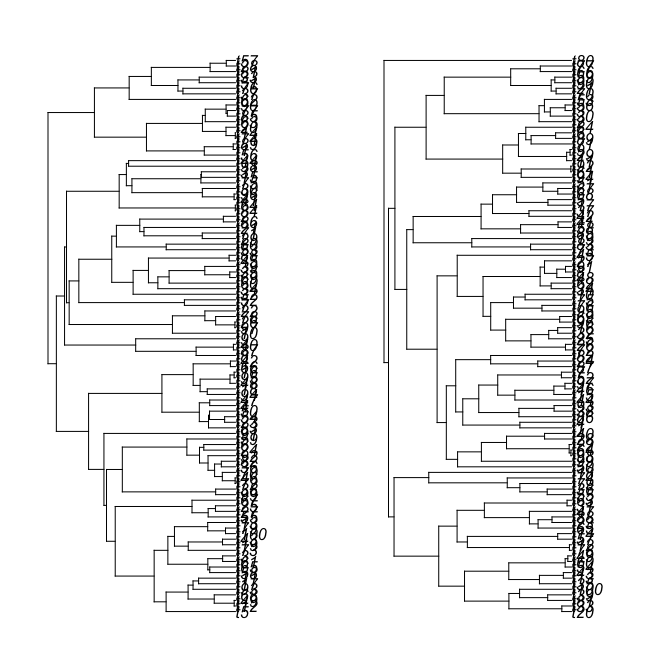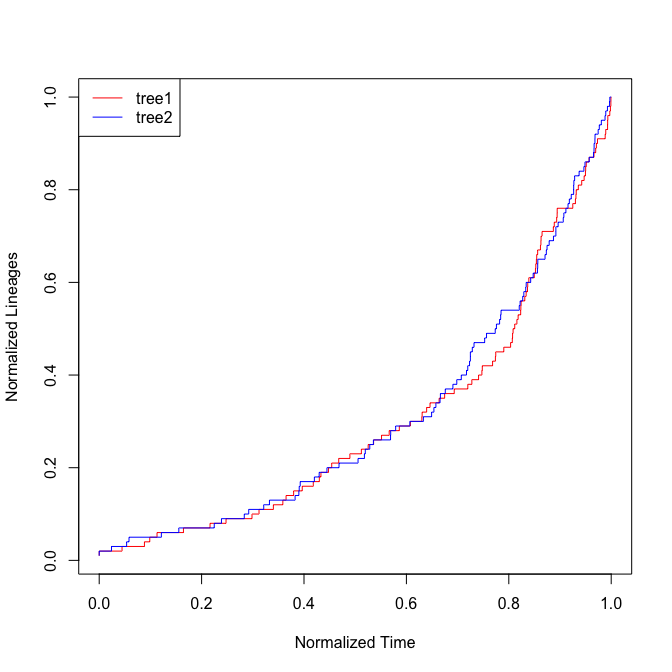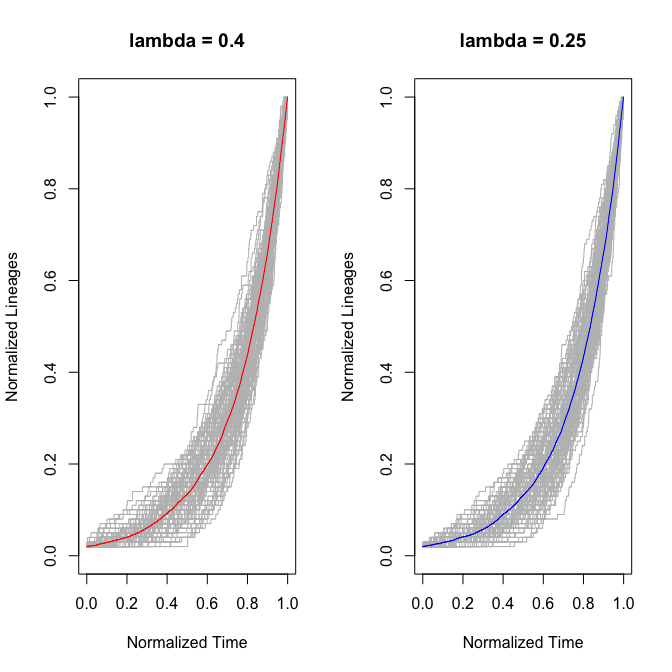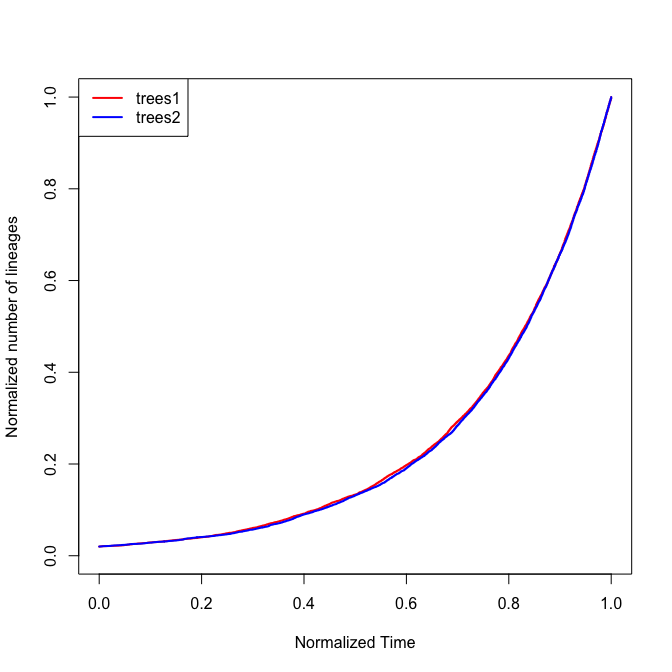# Walkthrough using the nLTT package

## nLTT statistic & nLTT plot

In order to provide some worked examples, we first generate two random phylogenetic trees, with similar parameter settings. We simulate one tree with lambda = 0.4, and one tree with lambda = 0.25, both with 100 tips.

    library(nLTT) #nolint
set.seed(42)
tree1 <- ape::rphylo(n = 100, birth = 0.4, death = 0.0)
tree2 <- ape::rphylo(n = 100, birth = 0.25, death = 0.0)
par(mfrow = c(1, 2))
par(mar = c(2, 2, 2, 2))
plot(tree1)
plot(tree2)The two trees look similar, but are not identical in shape.

This becomes even more obvious when we plot their normalized Lineage Through Time plots:

    nltt_plot(tree1, col = "red")
nltt_lines(tree2, col = "blue")
legend("topleft", c("tree1", "tree2"), col = c("red", "blue"), lty = 1)## Average nLTT matrix & Average nLTT plot

Sometimes, it might be more interesting to look at the average nLTT plot across a large number of replicate trees, or to calculate the average normalized Lineages Through Time, for a large number of replicate trees.

Let us first generate 100 random trees:

set.seed(42)
trees1 <- list()
trees2 <- list()
for (r in 1:100) {
trees1[[r]] <- ape::rphylo(n = 100, birth = 0.4, death = 0.0)
trees2[[r]] <- ape::rphylo(n = 100, birth = 0.25, death = 0.0)
}

Using the function nltts_plot we can now plot the normalized Lineages Through Time for all replicates, and on top of that plot the average normalized Lineages Through Time. The replicates are all plotted in grey, and the average lines are plotted in red and blue respectively:

par(mfrow = c(1, 2))
nltts_plot(trees1, dt = 0.001, plot_nltts = TRUE,
col = "red", main = "lambda = 0.4")
nltts_plot(trees2, dt = 0.001, plot_nltts = TRUE,
col = "blue", main = "lambda = 0.25")Instead of plotting the average normalized Lineages Through Time, we can also calculate them, and store them in a matrix:

m1 <- get_average_nltt_matrix(trees1, dt = 0.001)
m2 <- get_average_nltt_matrix(trees2, dt = 0.001)

This allows us to do further analysis, and also allows us to plot the two average nLTT plots on top of each other, to see whether the two average nLTT plots differ from each other (mind you, m1 and m2 are matrices, not nLTT objects, so we can plot them using the normal plotting tools available in R)

m1 <- get_average_nltt_matrix(trees1, dt = 0.001)
m2 <- get_average_nltt_matrix(trees2, dt = 0.001)
plot(m1, type = "s", col = "red", lwd = 2, xlim = c(0, 1), ylim = c(0, 1),
xlab = "Normalized Time", ylab = "Normalized number of lineages")
lines(m2, type = "s", col = "blue", lwd = 2)
legend("topleft", c("trees1", "trees2"), col = c("red", "blue"),
lty = 1, lwd = 2)